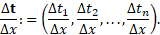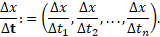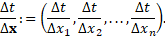iSoul In the beginning is reality.

# Category Archives: Knowing

epistemology, science, kinds of knowledge, methodology

# What is a clock?

What is a clock? it is a device that measures time, but what are the essentials of a clock? I submit these are the essentials of a clock:

(1) A clock requires a uniform motion. Because only the kinematics (not the dynamics) are significant, a uniform rotation is acceptable. But because the result will be represented as a line – a timeline or time axis – a linear uniform motion has a more direct connection with what is measured, so let us take the first essential as a uniform linear motion.

(2) In order for clocks to be measuring alike, it is necessary that there be a standard rate for all clocks. In addition, clocks should have a standardized beginning point, so that clocks are interchangeable.

(3) A clock requires a pointer which indicates the present time on a time scale as it moves at the standard uniform rate. This would be the hands and dial on a common analogue clock. On a linear clock it is a part whose position in motion is interpreted as the present value of time. The pointer and scale are essentials of a clock.

Furthermore, a clock must be interpreted as showing the present time of the observer’s rest frame.

All the essentials of a clock can be represented by a frame in standard uniform motion relative to the observer’s rest frame. In that case, a clock should be definable in terms of frames of reference: one rest frame and one frame in uniform motion relative to the observer’s rest frame, as in the following.

# Space, time, and dimension

The post continues the ones here, here, and here.

There are three dimensions of motion. The extent of motion in each dimension may be measured by either length or time (duration). There are three dimensions of length and three dimensions of time (duration) for a total of six dimensions.

But there is no six-dimensional metric. Why? Because a metric requires all dimensions to have the same units, which requires a ratio to convert one unit into the other unit. The denominator on a ratio is a one-dimensional quantity, which means either the length or time dimensions need to be reduced by two dimensions.

This ratio is a conversion factor that is either a speed, which multiplied by a time equals a length, or a pace, which multiplied by a length equals a time. In general a speed is the ratio Δdr²/|Δdt²| = Δdr²/(Δt1² + Δt2² + Δt3²)1/2, and a pace is the ratio Δdt²/|Δdr²| = Δdt²/(Δx² + Δy² + Δz²)1/2. The denominator is a distance or distime, which is a linear measure of length or time (duration).

The conversion factors required are the speed of light in a vacuum, c, or its inverse, the pace of light in a vacuum, k. The resulting four-dimensional metric is either c²dt² − dx² − dy² − dz² (with time reduced to one dimension) or dt1² − dt2² − dt3² − k²dr² (with space reduced to one dimension).

These metrics are often simplified by taking c = 1 and k = 1 so that symbolically they are the same. Their units are not the same, however.

Each metric may be further reduced by separating space and time, as in classical physics. Then the space metric is |Δdr²| = (Δx² + Δy² + Δz²)1/2 and the time metric is |Δdt²| = (Δt1² + Δt2² + Δt3²)1/2. In the classical (3+1) of three space dimensions and one time dimension, time is replaced by its metric, and in the classical (1+3) of one space dimension and three time dimensions, space is replaced by its metric.

# Space and time as frames

An observer is a body capable of use as a measurement apparatus. An inertial observer is an observer in inertial motion, i.e., one that is not accelerated with respect to an inertial system. An observer here shall mean an inertial observer.

An observer makes measurements relative to a frame of reference. A frame of reference is a physical system relative to which motion and rest may be measured. An inertial frame is a frame in which Newton’s first law holds (a body either remains at rest or moves in uniform motion, unless acted upon by a force). A frame of reference here shall mean an inertial frame.

A rest frame of observer P is a frame at rest relative to P. A motion frame of observer P is a frame in uniform motion relative to P. Each observer has at least one rest frame and at least one motion frame associated with it. An observer’s rest frame is three-dimensional, but their motion frame is effectively one-dimensional, that is, only one dimension is needed.

Space is the geometry of places and lengths in R3. A place point (or placepoint) is a point in space. The space origin is a reference place point in space. The location of a place point is the space vector to it from the space origin. Trime (3D time) is the geometry of times and durations in R3. A time point (or timepoint) is a point in trime. The time origin is a reference time point in trime. The chronation of a time point is the trime vector to it from the time origin.

A frame of reference is unmarked if there are no units specified for its coordinates. A frame of reference is marked by specifying (1) units of either length or duration for its coordinates and (2) an origin point. A space frame of observer P is a rest frame of P that is marked with units of length. A time frame of observer P is a motion frame of P that is marked with units of duration.

Speed, velocity, and acceleration require an independent motion frame. Pace, lenticity, and retardation require an independent rest frame. These independent frames are standardized as clocks or odologes so they are the same for all observers.

Let there be a frame K1 with axes a1, a2, and a3, that is a rest frame of observer P1, and let there be a motion frame K2 with axes 1, 2, and 3, that is a motion frame of P1 along the coincident a1-a´1 axis. See Figure 1.

# Vectors and Functions in Space and Time

The time velocity of an n-dimensional vector variable or vector-valued function Δx per unit of an independent scalar variable t equalsSimilarly, the space lenticity with Δt and Δx, respectively:The rate of a scalar variable Δx per unit of an independent n-dimensional vector variable or vector-valued function Δt equalsThe same except with Δt and Δx, respectively:# Galilean decompositions of the Lorentz transformation

The background for this post is here.

For space with time (3+1):

The gamma transformation (matrix Γ) expresses the time dilation of clocks and length contraction of rods with a relative speed:

Use vector (t  x)T. The gamma transformation is conjugate to the Lorentz boost (matrix Λ) by the Galilean transformations (G, GT), i.e., GTΓG = Λ:

or

This expands to

The matrix second from the right represents the Tangherlini transformation (or inertial synchronized Tangherlini transformation).

For time with space (1+3):

# Lorentz transformation derivations

What follows are four derivations of the Lorentz transformation from the complete Galilei (Galilean) transformations in space with time (3+1) and time with space (1+3). Their intersection is linear space and time (1+1), which is the focus of the derivations. The other dimensions may be reached by rotations in space or time.

I. Space with Time (3+1)

Consider two inertial frames of reference O and O′, assuming O to be at rest while O′ is moving with velocity v with respect to O in the positive x-direction. The origins of O and O′ initially coincide with each other. A light signal is emitted from the common origin and travels as a spherical wave front. Consider a point P on a spherical wavefront at a distance x and x′ from the origins of O and O′ respectively. According to the second postulate of the special theory of relativity the speed of light, c, is the same in both frames, so for the point P:

x = ct, and x′ = ct′.

A. Time velocity

Define velocity v as the time velocity vt = ds/dt. Consider the standard Galilean transformation of ct and x with a factor γ, which is to be determined and may depend on β, where β = v/c:

x′ = γ(x − vt) = γ(x − βct) = γx(1 − β).

The inverse transformation is the same except that the sign of β is reversed:

x = γ(x′ + vt′) = γ(x + βct) = γx′(1 + β).

# Home is the horizon

As there is an inverse or harmonic algebra, so there is an inverse geometry, an inverse space. Home, the origin, is the horizon, the ends of the earth, and beyond that, the celestial equator, the heavens. We may attempt to journey to the centre of the earth with Jules Verne, but we’ll never make it because it is infinitely far away. We cannot plumb the ultimate depths within, the deep well of the heart. At the centre of it all is a bottomless pit, the hell of eternal darkness.The geometric inverse is with respect to a circle or sphere:

P’ is the inverse of P with respect to the circle. The inverse of the centre is the point at infinity.

The order of events in this geometry is their distance from the horizon, not the centre. The return to home is the end of events, the final event. The later the event, the better, since it is closer to the end, to home.

The destination is where we’ve come from and where we return. It is a round trip, a circuit, a cycle of life and change.

What we call the beginning is often the end
And to make an end is to make a beginning.
The end is where we start from. T. S. Eliot, Little Gidding

# Lorentz factor from light clocks

Space and time are inverse perspectives on motion. Space is three dimensions of length. Time is three dimensions of duration. Space is measured by a rigid rod at rest, whereas time is measured by a clock that is always in motion relative to itself.

This is illustrated by deriving the Lorentz factor for time dilation and length contraction from light clocks. The first derivation is in space with a time parameter and the second is in time with a space parameter (placepoint).

The first figure above shows frame S with a light clock in space as a beam of light reflected back and forth between two mirrored surfaces. Call the height between the surfaces that the light beam travels distance h. Let one time cycle Δt = 2h/c or h = cΔt/2, with speed of light c, which is the maximum speed.

The second figure shows frame with the same light clock as observed by someone moving with velocity v relative to S. Call the length of each half-cycle d, and call the length of the base of one cycle in space b.

# Proper and improper rates

The independent quantity in a proper rate is the denominator. The independent quantity in an improper rate is the numerator. If a rate is multiplied by a quantity with the units of the independent quantity and the result has the units of the dependent quantity, it is proper. Otherwise, it is improper.

A proper rate becomes improper if the proper rate is inverted. An improper rate becomes proper if the improper rate is inverted. If two or more improper rates are added, each must first be inverted. The result of adding proper rates must be inverted again to return to the original improper rate. This is harmonic addition:

If the addition of improper rates is divided by the number of addends so that it is the average or arithmetic mean of the inverted rates, then the result inverted is the harmonic mean:

Time speed is the speed of a body measured by the distance traversed in a known time, which is a proper rate because the independent quantity, time, is the denominator. Space speed is the speed of a body measured by the time it takes to transverse a known distance, which is an improper rate because the independent quantity, distance, is the numerator. Space speeds are averaged by the harmonic mean and called the space mean speed. The time mean speed is the arithmetic mean of time speeds.

Velocity normalized by the speed of light is proper because the invariant speed of light is independent. The speed of light divided by a velocity is improper and must be added harmonically. Lenticity normalized by an hypothesized maximum pace is proper, but if the lenticity is divided by the pace of light, it is improper.

# Space with time and their dual

For the first post in this series see here.

Space with time (3+1)

Space is that which is measured by length; time is that which is measured by duration. There are three dimensions of length and one dimension (or parameter) of duration. Direction in space is measured by an angle, which is part of a circle.

Spatial rates are dependent on another variable, usually interval of time (distime).

Time is that which is measured by duration. Events are ordered by time. Time as an independent variable decreases from the past to the present and increases from the present to the future.

Temporal rates are dependent on another variable, usually the interval of space (stance).

Dual: time with space (1+3)

The dual of space with time is time with space. The dual of space is time and the dual of time is space. Space corresponds to time and time corresponds to space.

Time is that which is measured by duration; space is that which is measured by length. There are three dimensions of duration and one dimension (or parameter) of length. Direction in time is measured by a turn, which is part of a rotation.

Temporal rates are dependent on another variable, usually interval of space (stance).

Space is that which is measured by length. Events are ordered by length (stance). Space as an independent variable decreases from a past there to here and increases from here to a future there.

Spatial rates are dependent on another variable, usually the interval of time (distime). Read more →